# 如何用简单的Python编写Web应用程序？Python的宗旨：简单胜于复杂，而Streamlit创建应用程序则完美地诠释了其易操作性。pip install streamlit

streamlit hello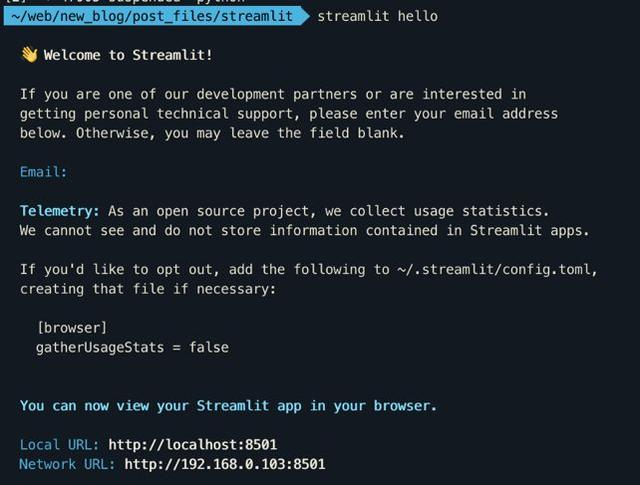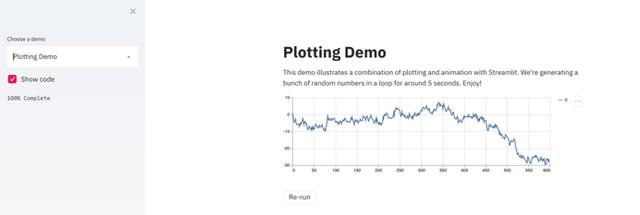Streamlit“你好，世界”

Streamlit旨在通过简单的Python代码实现简单的程序开发。

import streamlit as st

x = st.slider('x')

st.write(x, 'squared is', x * x)

streamlit run helloworld.py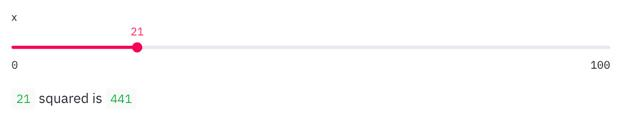• st.slider插件——滑动改变Web应用程序的输出内容。

• 以及通用的st.write 指令。笔者对于它能从图标、数据框和简单的文本中编写任何东西感到惊讶。后面会有详细介绍。Streamlit插件

1. 滑块

streamlit.slider(label, min_value=None, max_value=None, value=None, step=None, format=None)

2. 文本输入

import streamlit as st

url = st.text_input('Enter URL')

st.write('The Entered URL is', url)3. 复选框

import streamlit as st

import pandas as pd

import numpy as np

df = pd.read_csv("football_data.csv")

if st.checkbox('Show dataframe'):

st.write(df)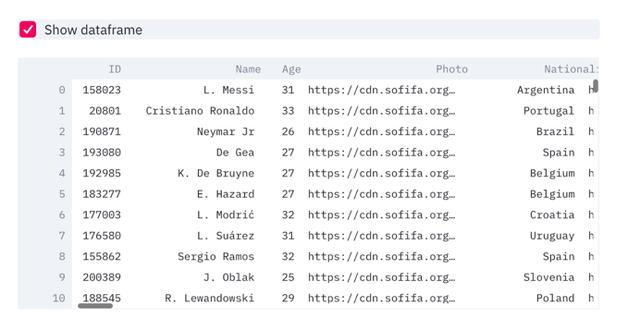4. 下拉框插件

import streamlit as st

import pandas as pd

import numpy as np

df = pd.read_csv("football_data.csv")option = st.selectbox(

'Which Club do you like best?',

df['Club'].unique())

'You selected: ', option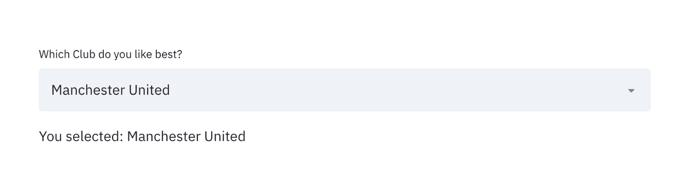5. 多选插件

import streamlit as st

import pandas as pd

import numpy as np

df = pd.read_csv("football_data.csv")

options = st.multiselect(

'What are your favorite clubs?', df['Club'].unique())

st.write('You selected:', options)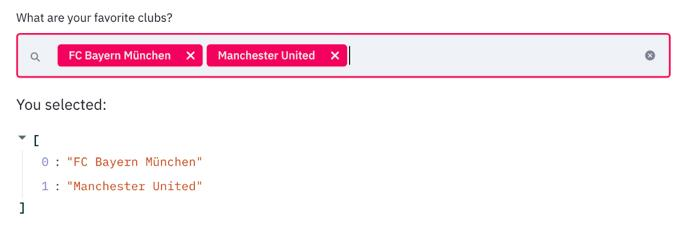import streamlit as st

import pandas as pd

import numpy as np

df = pd.read_csv("football_data.csv")

clubs = st.multiselect('Show Player for clubs?', df['Club'].unique())

nationalities = st.multiselect('Show Player from Nationalities?', df['Nationality'].unique())

# Filter dataframe

new_df = df[(df['Club'].isin(clubs)) & (df['Nationality'].isin(nationalities))]

# write dataframe to screen

st.write(new_df)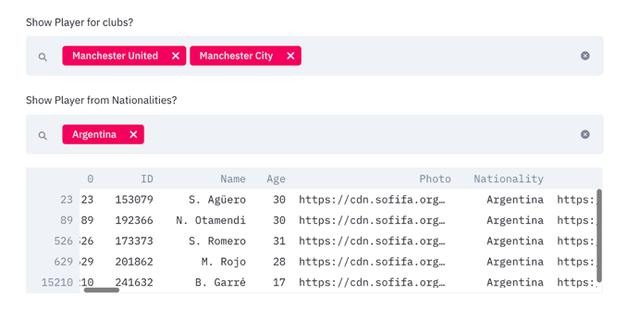Streamlit当前支持许多用于绘图的库，其中就有Plotly, Bokeh, Matplotlib, Altair和Vega图表。Plotly Express也可以，只不过没有详细说明。也存在一些内嵌式图表，相当于Streamlit“自带”的，比如st.line_chart 和st.area_chart.

import streamlit as st

import pandas as pd

import numpy as np

import plotly_express as px

df = pd.read_csv("football_data.csv")

clubs = st.multiselect('Show Player for clubs?', df['Club'].unique())

nationalities = st.multiselect('Show Player from Nationalities?', df['Nationality'].unique())new_df = df[(df['Club'].isin(clubs)) & (df['Nationality'].isin(nationalities))]

st.write(new_df)

# create figure using plotly express

fig = px.scatter(new_df, x ='Overall',y='Age',color='Name')

# Plot!

st.plotly_chart(fig)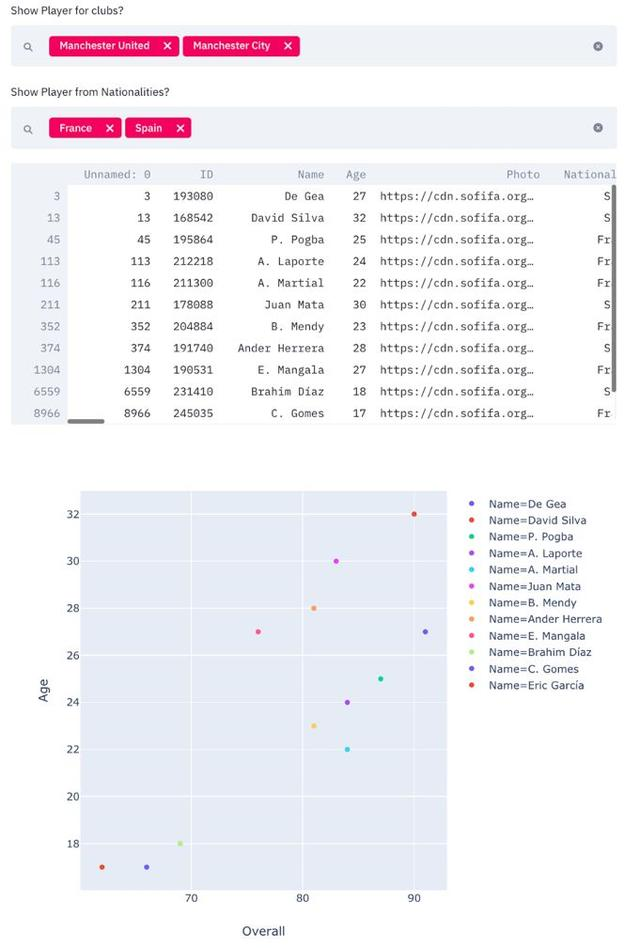1. 缓存

import streamlit as st

import pandas as pd

import numpy as np

import plotly_express as px

df = st.cache(pd.read_csv)("football_data.csv")

@st.cache

def complex_func(a,b):

DO SOMETHING COMPLEX

# Won't run again and again.

complex_func(a,b)

2. 侧边栏

import streamlit as st

import pandas as pd

import numpy as np

import plotly_express as px

df = st.cache(pd.read_csv)("football_data.csv")

clubs = st.sidebar.multiselect('Show Player for clubs?', df['Club'].unique())

nationalities = st.sidebar.multiselect('Show Player from Nationalities?', df['Nationality'].unique())

new_df = df[(df['Club'].isin(clubs)) & (df['Nationality'].isin(nationalities))]

st.write(new_df)

# Create distplot with custom bin_size

fig = px.scatter(new_df, x ='Overall',y='Age',color='Name')

# Plot!

st.plotly_chart(fig)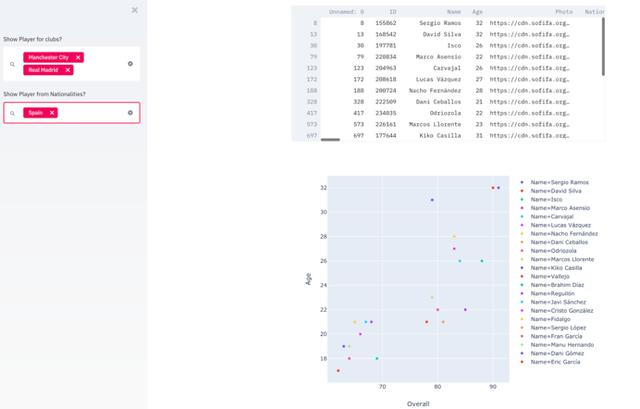3. Markdown标记语言可以吗？

import streamlit as st

import pandas as pd

import numpy as np

import plotly_express as px'''

# Club and Nationality App

This very simple webapp allows you to select and visualize players from certain clubs and certain nationalities.

'''

df = st.cache(pd.read_csv)("football_data.csv")

clubs = st.sidebar.multiselect('Show Player for clubs?', df['Club'].unique())

nationalities = st.sidebar.multiselect('Show Player from Nationalities?', df['Nationality'].unique())new_df = df[(df['Club'].isin(clubs)) & (df['Nationality'].isin(nationalities))]

st.write(new_df)

# Create distplot with custom bin_size

fig = px.scatter(new_df, x ='Overall',y='Age',color='Name')

'''

### Here is a simple chart between player age and overall

'''

st.plotly_chart(fig)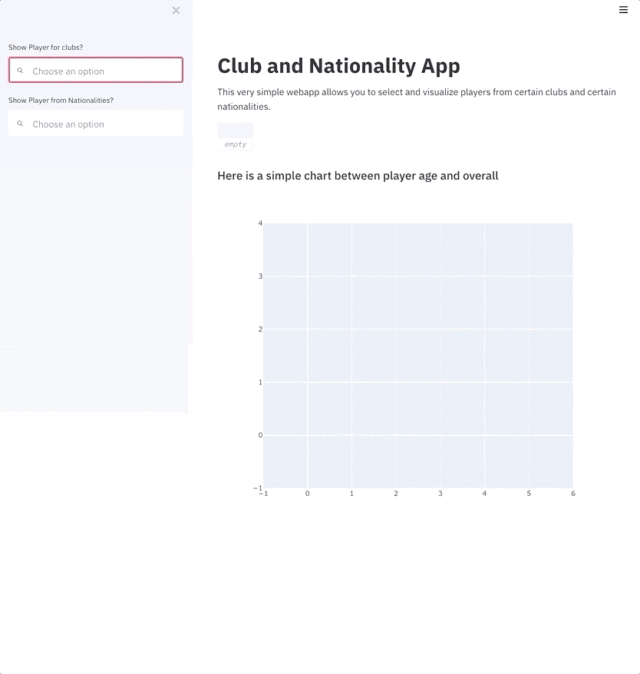Streamlit已经介绍了整个创建应用程序的步骤，笔者真没什么可补充的了。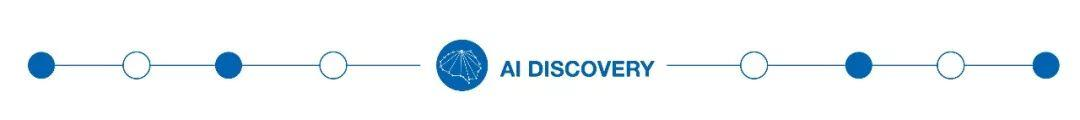（添加小编微信：dxsxbb，加入读者圈，一起讨论最新鲜的人工智能科技哦～）06-264万+
12-311万+
02-261万+
08-122481
10-257184
03-142万+
12-174986
10-26864
11-17341
12-08339
04-301456
09-287756
©️2020 CSDN 皮肤主题: 大白 设计师:CSDN官方博客